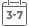# Bayesian Networks: An Introduction

• Publish Date: 2009-11-02
• Binding: Hardcover
• Author: Timo Koski;John Noble
• Sale
• \$132.46
• Regular price \$105.99Bayesian Networks: An Introduction provides a self-contained introduction to the theory and applications of Bayesian networks, a topic of interest and importance for statisticians, computer scientists and those involved in modelling complex data sets. The material has been extensively tested in classroom teaching and assumes a basic knowledge of probability, statistics and mathematics. All notions are carefully explained and feature exercises throughout.

Features include:

• An introduction to Dirichlet Distribution, Exponential Families and their applications.
• A detailed description of learning algorithms and Conditional Gaussian Distributions using Junction Tree methods.
• A discussion of Pearl's intervention calculus, with an introduction to the notion of see and do conditioning.
• All concepts are clearly defined and illustrated with examples and exercises. Solutions are provided online.

This book will prove a valuable resource for postgraduate students of statistics, computer engineering, mathematics, data mining, artificial intelligence, and biology.

Researchers and users of comparable modelling or statistical techniques such as neural networks will also find this book of interest.

MORE FROM THIS COLLECTION